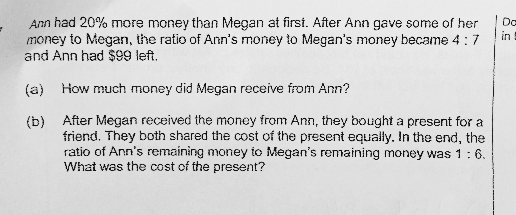# QuestionAnyone help me with attached question

Thanks

 Megan Ann Total At first 100u 120% × 100u = 120u 220u Ann gave Megan some money In the end, Ann:Megan = 4:7 (ie. Ann had 4/11, Megan 7/11) 7/11 × 220u = 140u 4/11  × 220u = 80u 220u Difference +40u -40u

80u = \$99

40u = 99 ÷ 2 = \$49.50

(a) Ann gave Megan \$49.50

Megan had 140u = 99 × 140 / 80 = \$173.25

Cost of present -> 2p

In the end

Ann -> \$99 – p

Megan -> \$173.25 – p

Given: Ann : Megan = 1 : 6

6(99 – p) = (173.25 – p)

594 – 6p = 173.25 – p

5p = 420.75

2p = 420.75 × 2 / 5 = \$168.30

(b) Cost of present -> \$168.30

Check

80u = 99

u = 1.2375

 Megan Ann At first 100u = 123.75 120u = 148.50 Ann gave Megan \$49.50 +49.50 -49.50 In the end 173.25 99

99 : 173.25 = 4 : 7 (checked)

Cost of present -> \$168.30

Each person’s share -> \$168.30 / 2 = \$84.15

 Megan Ann At first 173.25 99 Paid for present -84.15 -84.15 In the end 89.10 14.85

14.85 : 89.10 = 1 : 6 (checked)

Checked

0 Replies 1 Like

(a)
At first —
Ann : 1.2u
Megan : 1u

After —
Ann : (4/11) x 2.2 = 0.8u ——- 99
Megan : (7/11) x 2.2 = 1.4u

1u ——- 99/0.8 = 123.75
1.4u – 1u = 0.4u ——- 0.4 x 123.75 = 49.50

(b)
cost of the present ——- 2p
(0.8u – 1p) x 6 = 4.8u – 6p ——- 1.4u – 1p
1p ——- (4.8u – 1.4u)/5 = 0.68u = 0.68 x 123.75 = 84.15
2p ——- 2 x 84.15 = 168.30

Ans : (a) \$49.50; (b) \$168.30

0 Replies 0 Likes